Electron. J. Diff. Eqns., Vol. 2008(2008), No. 156, pp. 1-14.

### N-body problem in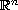: Necessary conditions for a constant configuration measure K. Zare

Abstract:
A formulation of the N-body problem is presented in which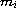and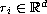are the mass and the position vector of the i-th body,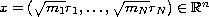and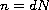(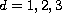). The configuration measure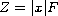, where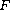is the Poincare's force function, which plays an important role in this formulation. The orbit plane is a two dimensional linear subspace ofspanned by the position vectorand the velocity vector. The N-body motion inhas been decomposed into an orbit in the orbit plane and the instantaneous orientation of the orbit plane. For a solution to stay on a level manifold of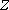, it is necessary that the orbit in the orbit plane be elliptic (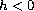), parabolic (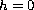) or hyperbolic (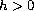) where h is the total energy. The instantaneous orientation of the orbit plane can be obtained by integration of certain differential equations. These possible solutions include the central configuration solutions in which the orbit plane is fixed in.

Submitted June 4, 2008. Published November 20, 2008.
Math Subject Classifications: 70F10.
Key Words: Configuration measure; Saari's conjecture; central configurations; generalized vector product; generalized momentum and eccentricity vectors.

Show me the PDF file (255 KB), TEX file, and other files for this article.Kal Zare Department of Mathematics Texas state University 601 University Drive San Marcos, TX 78666, USA email: kz11@txstate.edu